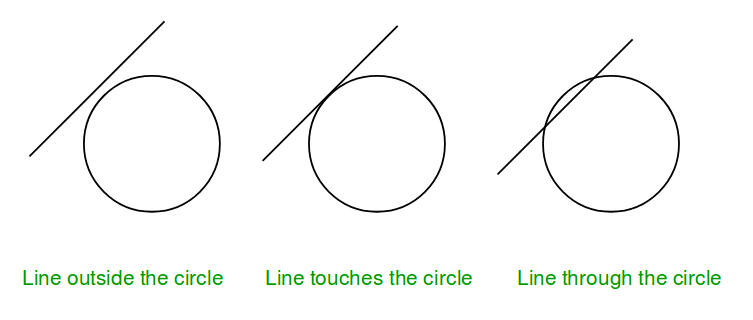# Check if a line touches or intersects a circle

Given coordinate of the center and radius > 1 of a circle and the equation of a line. The task is to check if the given line collide with the circle or not. There are three possibilities :

1. Line intersect the circle.
2. Line touches the circle.
3. Line is outside the circle.Note: General equation of a line is a*x + b*y + c = 0, so only constant a, b, c are given in the input.

Examples :

Input : radius = 5, center = (0, 0),
a = 1, b = -1, c = 0.
Output : Intersect

Input :  radius = 5, center = (0, 0),
a = 5, b = 0, c = 0.
Output : Touch

Input : radius = 5, center = (0, 0),
a = 1, b = 1, c = -16.
Output : Outside


## Recommended: Please try your approach on {IDE} first, before moving on to the solution.

The idea is to compare the perpendicular distance between center of circle and line with the radius of the circle.

Algorithm:
1. Find the perpendicular (say p) between center of circle and given line.
2. Compare this distance p with radius r.
……a) If p > r, then line lie outside the circle.
……b) If p = r, then line touches the circle.
……c) If p < r, then line intersect the circle.

How to find the perpendicular distance ?
Distance of a line from a point can be computed using below formula:Refer Wiki for details of above formula.

## C++

 // CPP program to check if a line touches or   // intersects or outside a circle.  #include  using namespace std;     void checkCollision(int a, int b, int c,                     int x, int y, int radius)  {      // Finding the distance of line from center.      int dist = (abs(a * x + b * y + c)) /                        sqrt(a * a + b * b);         // Checking if the distance is less than,       // greater than or equal to radius.      if (radius == dist)          cout << "Touch" << endl;      else if (radius > dist)          cout << "Intersect" << endl;      else         cout << "Outside" << endl;  }     // Driven Program  int main()  {      int radius = 5;      int x = 0, y = 0;      int a = 3, b = 4, c = 25;      checkCollision(a, b, c, x, y, radius);      return 0;  }

## Java

 // Java program to check if a line touches or   // intersects or outside a circle.     import java.io.*;     class GFG {             static void checkCollision(int a, int b, int c,                                  int x, int y, int radius)      {          // Finding the distance of line from center.          double dist = (Math.abs(a * x + b * y + c)) /                           Math.sqrt(a * a + b * b);                 // Checking if the distance is less than,           // greater than or equal to radius.          if (radius == dist)              System.out.println ( "Touch" );          else if (radius > dist)              System.out.println( "Intersect") ;          else             System.out.println( "Outside") ;      }             // Driven Program      public static void main (String[] args)       {          int radius = 5;          int x = 0, y = 0;          int a = 3, b = 4, c = 25;          checkCollision(a, b, c, x, y, radius);             }  }     // This article is contributed by vt_m.

## Python3

 # python program to check if a line  # touches or  intersects or outside  # a circle.     import math     def checkCollision(a, b, c, x, y, radius):             # Finding the distance of line       # from center.      dist = ((abs(a * x + b * y + c)) /             math.sqrt(a * a + b * b))         # Checking if the distance is less       # than, greater than or equal to radius.      if (radius == dist):          print("Touch")      elif (radius > dist):          print("Intersect")      else:          print("Outside")     # Driven Program  radius = 5 x = 0 y = 0 a = 3 b = 4 c = 25 checkCollision(a, b, c, x, y, radius)     # This code is contributed by Sam007

## C#

 // C# program to check if a line touches or   // intersects or outside a circle.  using System;     class GFG {             static void checkCollision(int a, int b, int c,                               int x, int y, int radius)      {          // Finding the distance of line from center.          double dist = (Math.Abs(a * x + b * y + c)) /                           Math.Sqrt(a * a + b * b);                 // Checking if the distance is less than,           // greater than or equal to radius.          if (radius == dist)              Console.WriteLine ("Touch");          else if (radius > dist)              Console.WriteLine("Intersect");          else             Console.WriteLine("Outside");      }             // Driven Program      public static void Main ()       {          int radius = 5;          int x = 0, y = 0;          int a = 3, b = 4, c = 25;                     checkCollision(a, b, c, x, y, radius);             }  }     // This article is contributed by vt_m.

## PHP

  $dist)   echo "Intersect";   else  echo "Outside" ;  }    // Driver Code  $radius = 5;  $x = 0;  $y = 0;  $a = 3;  $b = 4;  $c = 25;  checkCollision($a, $b, $c, $x, $y, \$radius);     // This code is contributed by Sam007  ?>

Output :

Touch


This article is contributed by Anuj Chauhan. If you like GeeksforGeeks and would like to contribute, you can also write an article using contribute.geeksforgeeks.org or mail your article to contribute@geeksforgeeks.org. See your article appearing on the GeeksforGeeks main page and help other Geeks.

Attention reader! Don’t stop learning now. Get hold of all the important DSA concepts with the DSA Self Paced Course at a student-friendly price and become industry ready.

My Personal Notes arrow_drop_up

Improved By : Sam007

Article Tags :
Practice Tags :

1

Please write to us at contribute@geeksforgeeks.org to report any issue with the above content.